• Call Now

1800-102-2727•

# Magnetic field - Laws, different cases, Practice problems, FAQs

Magnets find applications from small toys to heavy trains. The properties of magnets to be able to attract ferrous metals intrigues those who witness it for the first time. The earliest known form of magnet is the lodestone. The Earth we inhabit is a huge magnet. Experiments by Oersted proved that magnetism exists where current exists as well. The converse is also true, current can also be produced when magnetism exits. The latter is called “electromagnetism” and was proved by Michael Faraday, who produced current by moving a magnet near a coil. Moving charges generate current, which in turn generate magnetic fields. In this article, we will explore magnetic fields in detail.

• Magnetic field
• Biot Savart Law
• Magnetic field due to a straight conductor
• Magnetic field due to a circular coil
• Direction of magnetic field
• Force on a charged particle in a magnetic field
• Practice problems
• FAQs

## Magnetic field

Hans Christian Oersted, a Danish Physicist, placed a magnetic compass inside a current carrying loop . He noticed that the magnetic compass showed deflection whenever the current in the loop was varied. When the direction of the current is reversed, the deflection occurs in the opposite direction. This led him to the conclusion that there is a relation between current and magnetism. In other words, moving charges (which create current) caused magnetism in the conductor, which in turn deflected the compass.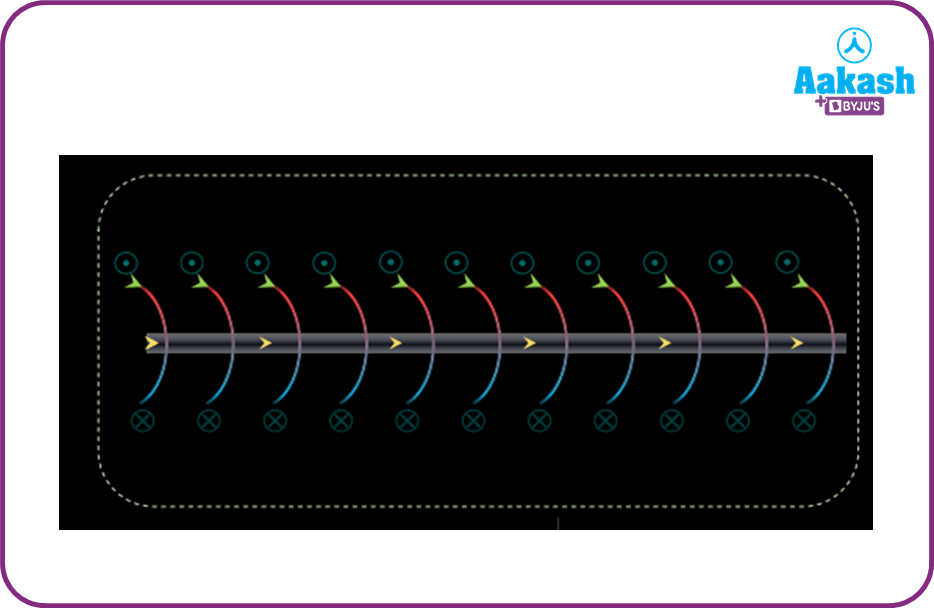The above diagram shows the magnetic field lines around a conductor. The cross sign indicates that the magnetic field is going into the plane of the paper while the dot sign indicates that the magnetic field is coming out of the plane of paper.

The magnetic field lines around a straight conductor resemble closed circular loops.

## Biot Savart Law

Let us consider a straight conductor in which the current flowing is I. The current element Idl is responsible for the magnetic field dB at the point P. The line drawn to P from the wire subtends an angle $\theta .$  Then according to Biot Savart law,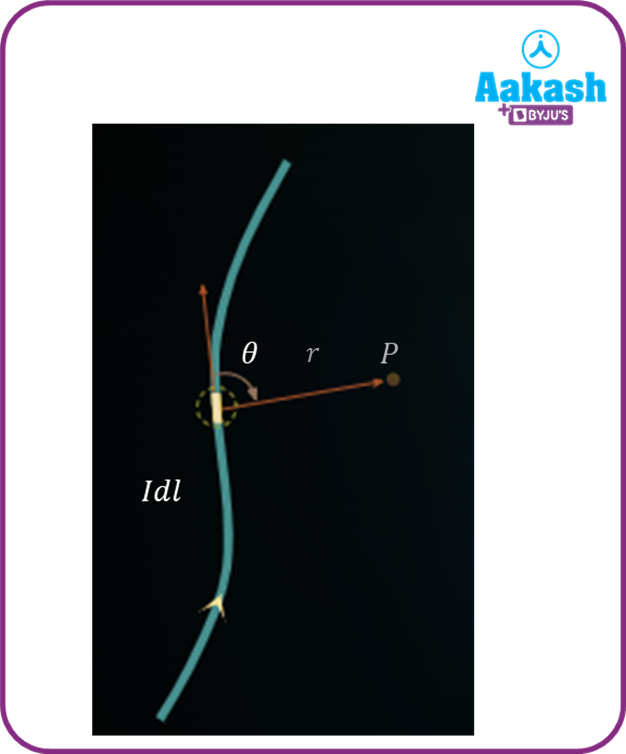dB=

Here r- distance of point P from the conductor

is called the permeability of free space or vacuum.

The SI unit of magnetic field is Tesla. Its dimensional formula is

## Magnetic field due to a straight conductor

Let us consider a finite wire in which the current element Idl is responsible for the magnetic field. The lines drawn from point P to the ends of the conductor make angles ${\varphi }_{1}$ and ${\varphi }_{2}$ respectively. Then,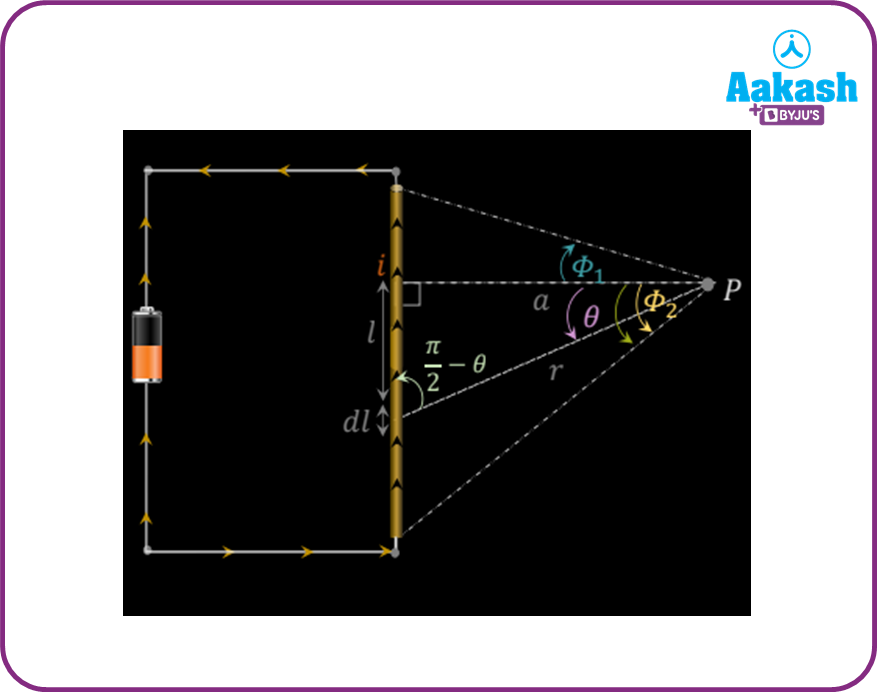dB=

Where ${\varphi }_{1}$ and ${\varphi }_{2}$ are the angles made by the line joining P with the extreme ends of the wire. From the above figure,

$cos\theta =$ $\frac{a}{r}$ and $\mathrm{tan}\theta =$ $\frac{l}{a}$ $l=atan\theta ;$

$d\theta$

$B=\frac{{\mu }_{0}I}{4\pi a}{\left[\mathrm{sin}\theta \right]}_{-{\varphi }_{2}}^{{\varphi }_{1}}⇒B=\frac{{\mu }_{0}I}{4\pi a}\left[\mathrm{sin}{\varphi }_{1}+\mathrm{sin}{\varphi }_{2}\right]$

For an infinitely long conductor

$⇒B=\frac{{\mu }_{0}I}{4\pi a}\left[1+1\right]=B=\frac{{\mu }_{0}I}{2\pi a}$

## Magnetic field due to a circular coil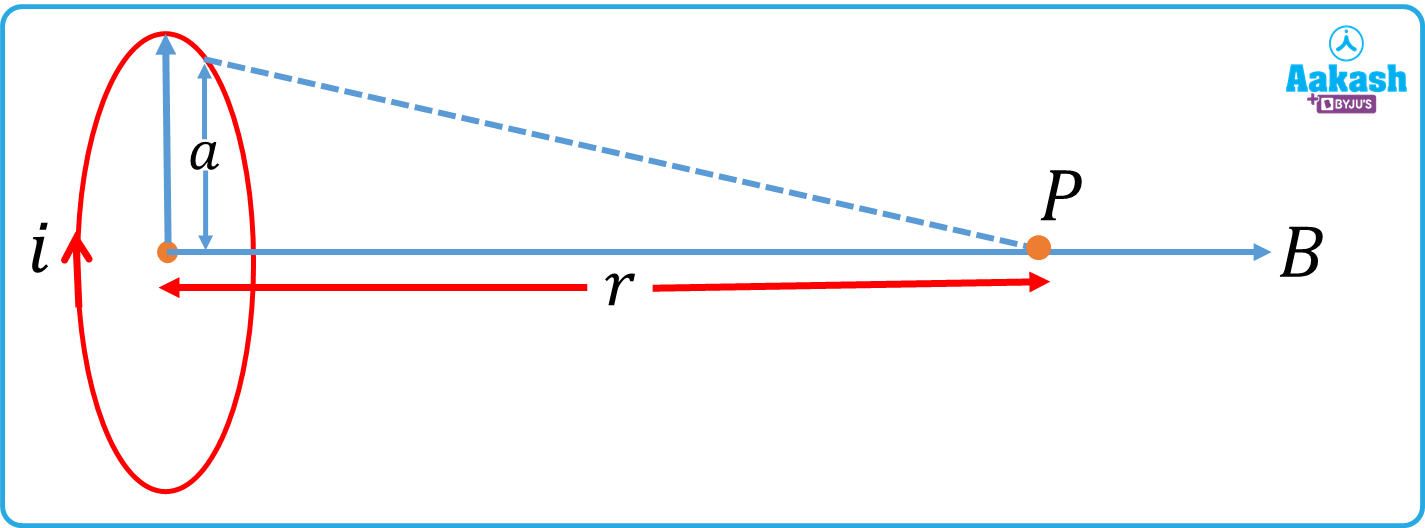Let us consider a circular coil having radius a. A point P is located at a distance r from the center of the coil. The magnetic field at this point on the axis of the coil due to the wire is,

B=

At the center of the coil, r=0

Here N is the number of turns in the coil.

## Direction of magnetic field

The direction of the magnetic field is given by the Right Hand Thumb Rule. Curl the four fingers of the right hand around the conductor, then the thumb points in the direction of the magnetic field. In the diagram shown below, the four fingers point outward, meaning the magnetic field is coming out of the plane of the paper(on the left of the conductor) but into the plane of the paper, on the right hand side of the conductor.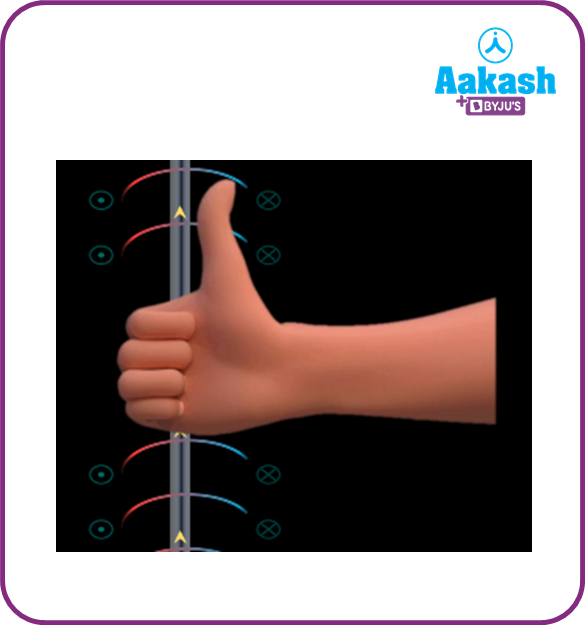## Force on a charged particle in a magnetic field

Let us consider a particle having charge q being shot into a magnetic field of strength $\stackrel{\to }{B}$ with a velocity $\stackrel{\to }{v}.$. Then the force exerted by the magnetic field on the charge would be,

$\stackrel{\to }{F}=q\left(\stackrel{\to }{v}×\stackrel{\to }{B}\right).$. This is called the Lorentz force.

In scalar form,

, where $\theta$ is the angle between $\stackrel{\to }{v}$ and $\stackrel{\to }{B}.$. It is clear that the force would be maximum if the angle between $\stackrel{\to }{v}$ and $\stackrel{\to }{B}.$ is ${90}^{0}.$.

Video explanation

From 11:32 to 52:05

## Practice problems

Q1.The magnetic field at center P would be,

(a)$\frac{{\mu }_{0}}{4\pi }$                                                       (b)$\frac{{\mu }_{0}}{\pi }$
(c)$\frac{{\mu }_{0}}{2\pi }$                                                        (d)$4{\mu }_{0}\pi$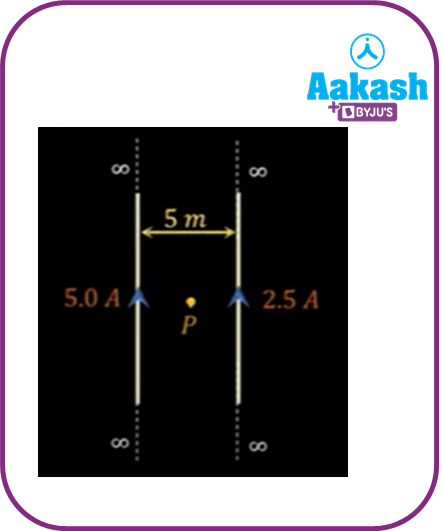Let B1 be the magnetic field due to wire 1(going into the plane of paper) and B2 be the magnetic field due to wire 2(directed out of the plane of paper). Then, the net magnetic field at P

${B}_{P}={B}_{1}-{B}_{2}$

${B}_{1}=\frac{{\mu }_{0}}{2\pi }×\frac{\left(5\right)}{\left(\frac{d}{2}\right)}$

${B}_{2}=\frac{{\mu }_{0}}{2\pi }×\frac{\left(2.5\right)}{\left(\frac{d}{2}\right)}$

d- distance between the wires.

${B}_{P}=\frac{2{\mu }_{0}\left(5\right)}{2\pi d}-\frac{2{\mu }_{0}\left(2.5\right)}{2\pi d}=\frac{2{\mu }_{0}}{2\pi \left(5\right)}\left(5-2.5\right)=\frac{{\mu }_{0}}{2\pi }$

Q2.Two concentric coils, each of radius r carrying currents I1 and I2 are placed perpendicular to each other. The net magnetic field at the center of the coils would be

(a)$\frac{{\mu }_{0}}{2\pi r}\sqrt{\left({{I}_{1}\right)}^{2}+\left({{I}_{2}\right)}^{2}}$                                        (b)$\frac{{\mu }_{0}\left({I}_{1}+{I}_{2}\right)}{2\pi r}$
(c)$\frac{{\mu }_{0}\left({I}_{1}-{I}_{2}\right)}{2\pi }$                                                       (d)None of these

The magnetic field due to the coils at the center are

${B}_{1}=\frac{{\mu }_{0}{I}_{1}}{2\pi r}$and ${B}_{2}=\frac{{\mu }_{0}{I}_{2}}{2\pi r}$

The net magnetic field at the center,

${B}_{center}=\sqrt{\left({{B}_{1}\right)}^{2}+\left({B}_{2}{\right)}^{2}}=\sqrt{{\left(\frac{{\mu }_{0}{I}_{1}}{2\pi r}\right)}^{2}+{\left(\frac{{\mu }_{0}{I}_{2}}{2\pi r}\right)}^{2}}=\frac{{\mu }_{0}}{2\pi r}\sqrt{\left({{I}_{1}\right)}^{2}+\left({{I}_{2}\right)}^{2}}$

Q3.The force exerted on a charged particle q which is traveling with a velocity v parallel to the magnetic field B would be

(a)qvB                                                     (b)$\frac{qvB}{2}$
(c) zero                                                   (d)None of these

A.c

The force

∴ F=0

Q4.A circular coil having radius R and N turns carries a current I. A point P is located at a distance x from the center of the coil at a distance $\sqrt{3}R$ from the center. The magnetic field at point P would be,

(a)                                                            (b)
(c)                                                        (d)

The magnetic field at the point P would be,

BP= here, $x=\sqrt{3}R$

BP=

## FAQs

Q1.What factors affect magnetism?
Magnetism is affected by heat, radiation and electrical currents. For instance, huge electrical currents in the vicinity generate their own magnetic field, disrupting the original magnetic field.

Q2.What gives rise to magnetism?
Mobile charges, when they start moving, generate electric current. The electric current in turn generates a magnetic field. Stronger the current, or faster the charges move, more would be the intensity of the magnetic field.

Q3.What creates Earth’s magnetism?
The molten lava inside the earth, combined with the rotation of the earth, gives rise to Earth’s magnetic field. The Earth’s magnetic field lines start from the North pole and end in the South pole.

Q4.What are some properties of magnetic field lines?
Here are some properties of magnetic field lines–1) they start from the north pole and end in the south pole. 2) They always form continuous closed lines. 3) The tangent drawn to the magnetic field line gives its direction. 4) Magnetic field lines never intersect

Q5.Where is the magnetic field strongest in a magnet?
In a bar magnet for example, the magnetic field lines start from the North pole and end in the south pole. The field lines appear close together near the poles, hence, it is strongest near the poles.Talk to our expert
Resend OTP Timer =
By submitting up, I agree to receive all the Whatsapp communication on my registered number and Aakash terms and conditions and privacy policy